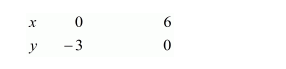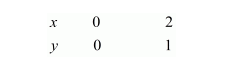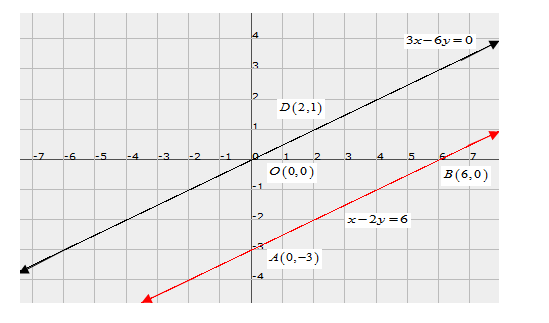# Solve the following systems of equations graphically:`
Question:

Solve the following systems of equations graphically:

$x-2 y=6$

$3 x-6 y=0$

Solution:

The given equations are:

$x-2 y=6 \quad \ldots \ldots .(i)$

$3 x-6 y=0 \quad \ldots \ldots . .(i i)$

Putting $x=0$ in equation $(i)$, we get:

$\Rightarrow 0-2 y=6$

$\Rightarrow y=-3$

$x=0, \quad y=-3$

Putting $y=0$ in equation $(i)$ we get:

$\Rightarrow x-2 \times 0=6$

$\Rightarrow y=6$

$x=6, \quad y=0$

Use the following table to draw the graph.Plotting the two points $A(0,-3)$ and $B(6,0)$ equation $(i)$ can be drawn.

Graph of the equation. (ii):

$3 x-6 y=0 \quad \ldots \ldots \ldots(i i)$

Putting $x=0$ in equation $(i i)$, we get:

$\Rightarrow 3 \times 0-6 y=0$

$\Rightarrow y=0$

$x=0, \quad y=0$

Putting $x=2$ in equation $(i i)$, we get:

$\Rightarrow 3 \times 2-6 y=0$

$\Rightarrow y=1$

$x=2, \quad y=1$

Use the following table to draw the graph.Draw the graph by plotting the two points $O(0,0)$ and $D(2,1)$ from table.We see that the two lines are parallel, so they won't intersect

Hence there is no solution